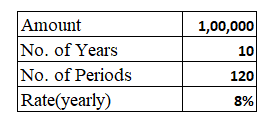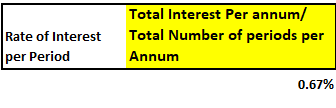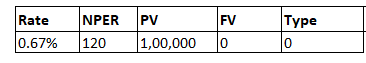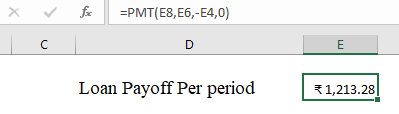#### How to Calculate Loan Payoff

Loan payoffs are simple calculations that are required every time you take a loan from a bank or a financial institution. To prevent yourself from being cheated by others or to be able to help others with their loans, follow the steps below for the loan payoff calculation.

## Loan data preparation

Input the details of the Loan Amount, number of years of loan repayment, Rate of Interest, and Period of Loan payment.

• Loan Amount: Principal amount borrowed
• Years of Loan repayment: Total no. of years in which loan is completely repaid.
• Rate of Interest: Per annum interest on the principal amount.
• Period: The span during which the loan payment is made (Ex: Each month, each quarter etc.).Here, No. of Periods = No. of Years * 12 (monthly repayment) = 10*12.

Note: You can choose a shorter loan amortization period, e.g., 6, 12 or 30 months instead of 10 years.

## Loan Payoff Calculations

Calculate the Rate of interest per period.

For the above figures, the rate of interest per period is = (8/12) % = 0.67%.## PMT Formula

Calculate the loan payoff per period using the Excel PMT formula.

=PMT( Rate, Nper, Pv, Fv, Type)

• Rate: Per period interest rate.
• Nper: Total no. of periods – Pv: Initial Value of the loan (has to be negative for PMT formula).
• Fv (optional): Fv stands for future value. If after paying off the last loan payment, you want to have any balance left it is the future value. Fv can be omitted (considered 0 if omitted).
• Type (optional):

0: If payment is to be done at end of period.

1: If payment is to be done at beginning of period.Loan payoff can be simply calculated by using the PMT formula and using the correct variables.Full formula is =PMT(E8,E6,-E4,0)

Calculate the loan balance:

Use the PV function to calculate the current loan balance. The syntax for the PV function is: =PV(rate, nper, pmt, [fv], [type]). For example, if you have made 12 payments on a 60-month loan, and the original loan amount was \$10,000 with an interest rate of 5%, the formula would be: =PV(5%/12, 48, -200, 10000). This will give you the current loan balance.

Calculate the payoff amount:

To calculate the loan payoff amount, simply add the current loan balance to any outstanding fees or charges. This will give you the total amount needed to pay off the loan.

Good luck with paying off your loans.